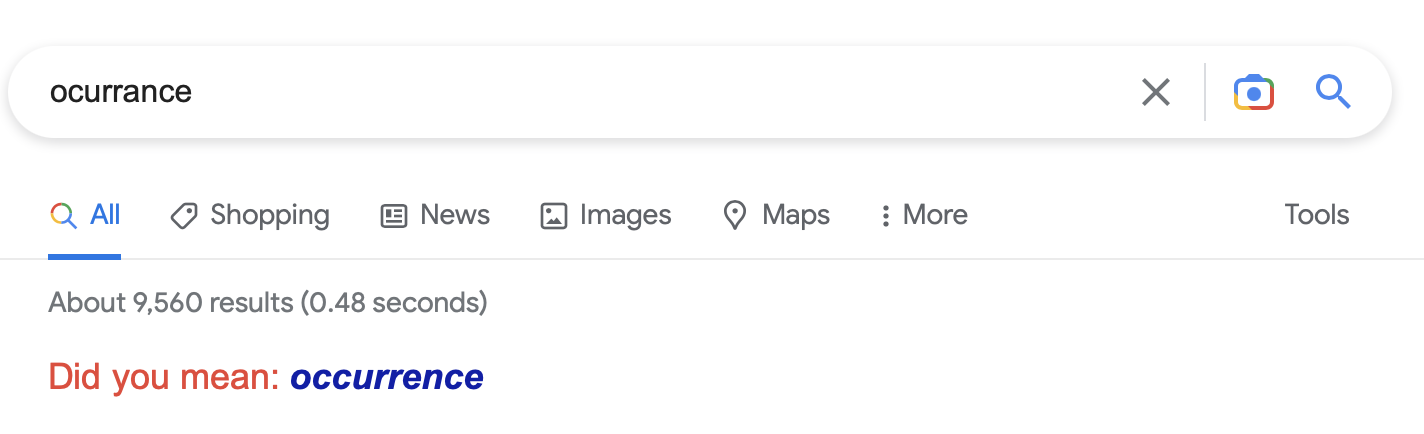# Lecture 25: Sequence Alignment

$\def\opt{ {\mathrm{opt}} }$

## Announcement

Homework 5 Posted

• 3 Questions
• Third question is challenge question

## Overview

1. Finishing Knapsack Problem
2. Sequence Alignment

## Knapsack Problem

Input:

1. A set $R$ of $n$ requests, each having
• duration (weight) $b_r$
• value $v_r$
2. Total time (weight) budget $B$

Output: A set $S$ of requests to service with

1. sum of durations of requests in $S$ is at most $B$
2. sum of values of requests is maximized

## A Recurrence Relation

Idea. keep track of remaining budget

• if $r_n$ is not serviced, remaining budget is $B$
• if $r_n$ is serviced, remaining budget is $B - b_n$

Definition. For $j = 0, 1, \ldots, n$, $\opt(j, C)$ is optimal value of set of requests from $1, 2, \ldots, j$ with budget $C$.

Recurrence relation:

$\opt(n, B) = \max(\opt(n - 1, B), v_n + \opt(n - 1, B - b_n))$

## Computing Optimal Values

Assume. All durations $b_i$ are integers at most $B$.

Compute. To compute $\opt(n, B)$:

• Generate a two dimensional array max where max[j, C] stores the value $\opt(j, C)$

Initialization. max[j, 0] <- 0 for all j

Apply Recursion Relation.

• max[j, C] <- Max(max[j-1, C], v[j] + max[j-1, C - b[j]])

## Pseudocode

  FindMax(R, n, B):
max <- new 2d array of dimensions n+1, B+1
set max[0, C] <- 0 for C = 0 to B
for j from 1 to n
(b, v) <- i-th request in R
for C from 0 to B
if b <= C then
max[j, C] <- Max(v + max[j-1, C-b], max[j-1,C])
else
max[j, C] <- max[j-1, C]
return max[n, B]


## Correctness

Claim. For all $j$ and $C$, $\max[j, C] = \opt(j, C)$

Proof. Induction on $j$.

Base case $j = 0$. Optimal subset of size $0$ has value $0$.

Inductive step $j \implies j+1$.

• suppose claim true for all $i \leq j$
• consider two possibilities:
1. request $j+1$ is in optimal subset $S$

$\opt(j+1, C) = v_{j+1} + \opt(j, C - b_{j+1}) = v_{j+1} + \max[j, C - b_{j+1}]$

2. request $j+1$ is not in optimal subset $S$

$\opt(j+1, C) = \opt(j, C) = \max[j, C]$

## Running Time?

  FindMax(R, n, B):
max <- new 2d array of dimensions n+1, B+1
set max[0, C] <- 0 for C = 0 to B
for j from 1 to n
(b, v) <- i-th request in R
for C from 0 to B
if b <= C then
max[j, C] <- Max(v + max[j-1, C-b], max[j-1,C])
else
max[j, C] <- max[j-1, C]
return max[n, B]


## Conclusion

For the knapsack problem with $n$ requests and budget $B$, we can find compute $\opt(n, B)$ in $O(B n)$ time.

• assuming the duration of each request is an integer

# Sequence Alignment## Question

How similar are the following strings?

## Hamming Distance

For how many indices do the strings disagree?

## (Dis)similarity and Alignment

How could we transform one string into the other?

## Optimal Alignment

Given two strings/arrays $X$ and $Y$ form a matching between characters

• matching $M$ is a set of pairs of matched indices

Rules for matching:

• each character is matched with at most one other character
• some characters may be unmatched
• matched characters cannot “cross”
• if $(i, j)$, $(i’, j’)$ are matched with $i < i’$, then $j < j’$

## Matching Penalties

Given a matching $M$ between strings $X$ and $Y$

• incur penalty $\delta$ if an index $i$ in $X$ or $Y$ is unmatched
• incur penality $\alpha$ if $(i, j)$ matched, $X[i] \neq X[j]$

Total penalty is sum of individual penalties

Example.

## Sequence Alignment Problem

Input:

• Sequences $X$ and $Y$ of characters of length $n$ and $m$, respectively
• Penalties $\delta, \alpha$ for omission/mismatch

Output:

• A matching $M$ between indices of $X$ and $Y$
• $M$ minimizes total penalty of matching

## An Observation

Suppose

• $X$ sequence of length $n$
• $Y$ sequence of length $m$
• $M$ a matching between $[1, n]$ and $[1, m]$nn

Claim. Then one of the following holds:

1. $(n, m)$ is in $M$
2. $n$ is unmatched in $M$
3. $m$ is unmatched in $M$

Why?

## A Recursive Solution?

Idea. Use previous claim to give recursive characterization of optimal alignment.

How?

Define

• $\opt(i, j) =$ minimum penalty of aligning $X[1..i]$ and $Y[1..j]$
• $M_{i,j}$ is minimum penalty matching between $X[1..i]$ and $Y[1..j]$
• by claim, there are three cases
1. $(i, j) \in M_{i, j}$
2. $i$ unmatched in $M_{i, j}$
3. $j$ unmatched in $M_{i, j}$

## Recursive Solution?

Question. What is a recurrence relation for $\opt(i, j)$?

## Iterative Solution

Construct a two dimensional array p[0..n, 0..m]

• p[i, j] should store $\opt(i, j)$

Question 1. How to initialize p?

Question 2. How to fill out p?

## Example

• $X = [R, I, T, E]$
• $Y = [T, I, E, R]$
• $\delta = \alpha = 1$

## Algorithm Pseudocode

  Alignment(X, Y, a, d):
p <- 2d array of dimension (n+1) x (m+1)
for i from 0 to n, p[i, 0] <- i * d
for j from 0 to m, p[0, j] <- j * d
for i from 1 to n
for j from 1 to m
unmatchX <- p[i-1, j] + d
unmatchY <- p[i,j-1] + d
match <- p[i-1,j-1]
if X[i] != Y[j] then match <- match + a
p[i, j] <- Min(unmatchX, unmatchY, match)
return p[n, m]


Running time?

## Conclusion

Optimal alignment between strings can be found in $O(n m)$ time where strings have lengths $n$ and $m$, respectively.

## Next Time

Shortest paths, revisited